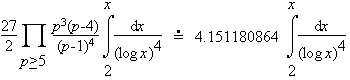A prime quadruple is four consecutive primes, such that the first and the last differ by 8, that look like (p, p+2, p+6, p+8). For technical reasons (see prime k-tuple) the case (3, 5, 7, 11) is omitted. The first few are as follows.

(5, 7, 11, 13), (11, 13, 17, 19), (101, 103, 107, 109), (191, 193, 197, 199), (821, 823, 827, 829), (1481, 1483, 1487, 1489), (1871, 1873, 1877, 1879), (2081, 2083, 2087, 2089), (3251, 3253, 3257, 3259), and (3461, 3463, 3467, 3469)

It is conjectured that there are infinitely many such primes. In fact the Hardy-Littlewood prime k-tuple conjecture suggests that the number less than x is approximatelyThe actual number less than 100,000,000 is 4768. The Hardy-Littlewood estimate above is 4734.